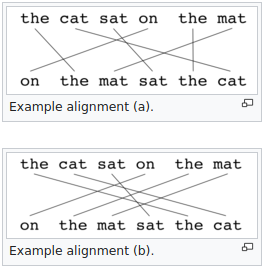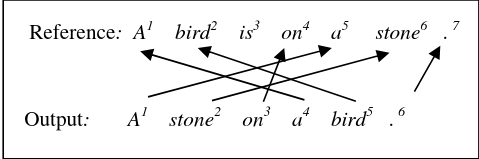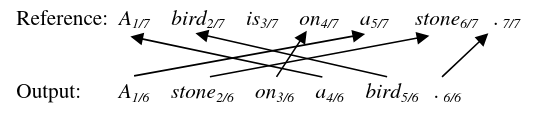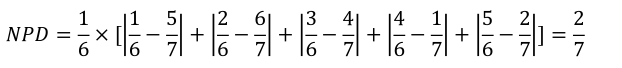Machine Translation(MT) is the use of a software to translate text or speech from one language to another. One of the issues in MT is how to evaluate the MT system to reasonably tell us whether the translation system makes an improvement or not. This is a challenge because unlike other machine learning problems where there can be only one correct answer, in MT, given a certain sentence in a particular language to translate, there could be multiple different translations that are equally good translations of that sentence which may vary in the choice of word or in the order of words and humans can clearly distinguish this. So how do we evaluate how good our machine translation model is given that there can be more than one correct translations of a particular sentence?

There have been traditional methods like human evaluations which are extensive but tend to be expensive, time-consuming and this involves human labor that cannot be reused. Researchers have gone ahead to create automatic evaluation methods for MT which provide quick and frequent evaluations which correlate highly with human evaluations. Some popular automatic evaluation methods include:

• BLEU Score
• NIST
• Word error rate(WER)
• METEOR
• LEPOR

In this article, we will look at some of the popular automatic evaluation metrics that are being used and how they differ from one another.

Here’s what we will cover:

1. Introduction
2. BLEU
3. METEOR
4. LEPOR
1. Design of the LEPOR metric
2. Precision and recall

# Introduction

One measures the quality of a translation based on how close it is to a professional human translation. So, the closer the machine translation is to a professional human translation, the better it is. To judge the quality of a machine translation, one measures how close it is to one or more professional human translations according to a numerical metric. These professional human translations are known as reference translations and are provided as part of the dev or test set. We will be looking at how some of these metrics are calculated and also identify the drawbacks of some of these metrics and why one is prefered over the other.

# BLEU

Bilingual Evaluation Understudy(BLEU) is one of the most popular metrics that’s being used to evaluate sequence to sequence tasks such as machine translation.

Let’s say we have a swahili sentence, its reference traslations, which are various correct ways the sentence can be translated to in to english and the MT output which are the outputs from our MT model.

Swahili : Kuna paka kwenye mkeka

Reference 1: The cat is on the mat
Reference 2: There is a cat on the mat

MT Output 1: the cat the cat on the mat
MT Output 2: the the the the the the the

The intuition behind the BLEU score is that a good translation shares many words and phrases with the references. Therefore, BLEU compares n-grams of the machine translation output with the n-grams of the reference translations, then count the number of matches, where the matches are position independent.

## Standard n-gram precision

BLEU metric is based on the presison metric. Precision is computed by counting up the number of machine translated words(n-grams) that occur in any reference translation and divide that by the total number of words in the candidate translation.

Using the example above, calcualting the unigram precision of MT output 1, we would count the number of unigrams in MT Output 1 that appear in any of the reference sentences then divide that total count with the total number of words in the machine translated sentence as shown below:

unigram shown in refrence?
the 1
cat 1
the 1
cat 1
on 1
the 1
mat 1
Total 7

Therefore, unigram precision = 7/7 = 1.0
If you do the same to MT Output 2, you will get a unigram precision of 8/8 = 1.0

As you can see from the results of MT Output 2, this is not a good measure because it says the MT Output has high precision, but that’s not the case.

To deal with this problem, they proposed a modified precision method.

## Modified n-gram precision

It is computed by:

• Counting the maximum number of times a word occurs in any single reference translation
• Clipping the total count of each MT output word by its maximum reference count
• Add all the clipped counts
• Divide the clipped counts by the total(unclipped) number of candidate words

Using our example from above, we would now end up with the table shown:

unigram clip count total
the 2 3
cat 1 2
on 1 1
mat 1 1
Total 5 7

Therefore our modified precision score now becomes: 5/7 = 0.714
. Compared to precision, we have seen that the modified precision is a better metric. It can also be computed the same way for any $n$ (bigram, trigram etc).

## BLEU Algorithm

BLEU is computed by combining a number of these modified n-gram precisions using the formula below:

$BLEU = BP.\exp\left(\sum_{n=1}^{N} w_n \log p_n\right)$

Where $$p_n$$ is the modified precision for $$n$$ gram, $$w_n$$ is the weight between 0 and 1 for $$log p_n$$ and $$\sum_{n=1}^{N} w_n = 1$$. The average logarithm with uniform weights is used because their experiments show that the modified n-gram precision decays exponentially with $$n$$: The unigram precision is much larger than modified bigram precision which is also larger than the modified trigram precision and so on. BP is the brevity penalty which is used to penalize short machine translations. The BP is said to be 1 when the candidate length is the same as any reference translation length and is penalized if the MT output is less than the reference text. $$c$$ represents the MT outputs and $$r$$ the reference texts. In the case of multiple reference sentences, $$r$$ is taken to be the sum of the lengths of the sentences whose lengths are closest to the lengths of the MT outputs.

$\begin{equation} BP=\begin{cases} 1, & \text{if  c > r}.\\ exp^{(1-\frac{c}{r})}, & \text{otherwise}. \end{cases} \end{equation}$

To produce a score for the whole corpus, the modified precision scores for the segments are combined using the geometric mean which is then multiplied by a brevity penalty.

To read more about BLEU, check out the paper: BLEU: a Method for Automatic Evaluation of Mahicne TRanslation

# METEOR

METEOR is based on generalized concept of unigram matching between the machine translations and human-produced reference translations unlike BLEU, which is based on matching n-gram translations. It was designed to explicitly address several observed weakenesses in the BLEU metric. Not only is METEOR able to match words that are identical but also words with identical stem and words that are synonyms of each other.

Once this generalized unigram matches between the two strings have been found, METEOR computes a score for this matching using a combination of unigram-precision, unigram-recall and a measure of how out-of-order the words of the MT output are with respect to the reference.

METEOR attempts to address several weaknesses that have been observed in BLEU such as:
The lack of recall - BLEU does not explicitly use recall but instead uses the Brevity Penalty. This, they believe does not adequately compensate for the lack of recall.
Use of Higher Order N-grams - BLEU uses higher order N-grams as an indirect measure of how well the translation is formed gramatically. They believe that checking the word order to measure level of grammaticality is a better account for the importance of grammaticality as a factor in the MT metric and result in better correlation with human judgements of translation quality.
Lack of Explicit Word-matching Between Translation and Reference -
Use of Geometric Averaging of N-grams- BLEU uses geometric averaging of n-grams which results in a score of zero whenever one component n-gram scores is zero

## METEOR metric

METEOR evaluates a translation by computing a score based on explicit word-to-word matches between the translation and a reference trasnlation. If more than one reference translation is available, the given translation is scored against each reference independently and the best score is reported.The alignment is a set mappings between a unigram in one string and a unigram in another string. Every unigram in the candidate translation must map to zero or one but not more than one unigram in the reference.If there are two alignments with the same number of mappings, the alignment is chosen with the fewest crosses, that is, with fewer intersections of two mappings. From the two alignments shown, alignment (a) would be selected at this point.

The alignment is incrementally produced through a series of stages, each stage consisting of two distinct phases

first phase A module lists all the posible unigram mappings between the two strings. Different modules map unigrams based on different criteria. The modules are as follows:

• exact module maps two unigrams if they are exactly the same, eg “computer” is mapped to “computer” but not “computers”.
• porter stem maps two unigrams if they are the stemmed using the porter stemmer eg, “computer” matches to both “computer” and “computers”.
• WN synonymy module maps two unigrams if they are synonyms of each other.

second phase The largest subset of these unigram mappings is selected such that the resulting set consitutes an alignment where each unigram maps to at mos one unigram in the other string.

Once all the stages have been run and a final alignment has been produced between the system translation and the reference translation, the METEOR score for this pair of translations is computed as follows:

## unigram precision (P)

Unigram precision (P) is computed as the ratio of the number of unigrams in the candidate translation that are also found in the reference translation to the number of unigrams in the candidate translation.

## Unigram recall (R)

Unigram recall (R) is computed as the ratio of the number of unigrams in the candidate translation that are also found in the reference translation to the number of unigrams in the reference translation.

## Fmean

Next the Fmean is calculated by combining the precision and recall through a harmoni-mean which places most of the weight on the recall(9 times more than precision)

$F_{mean} = \frac{10PR}{R + 9P}$

so far METOR is based on unigram matches, to take into account longer n-gram matches, METEOR computes a penalty for a given alignment. The penalty is computed as follows:

$Penalty = 0.5 * \left(\frac{chunks}{unigrams\_matched}\right)$

The unigrams are grouped into the fewest possible chunks where a chunk is defined as a set of unigrams that are adjacent in the candidate and in the reference. The longer the adjascent mappings between the candidate and the reference, the fewer chunks there are. A translation that is identical to the reference will give just one chunk.

Finally, the METEOR score for the given alignment is computer as follows:

$Score = Fmean*(1-Penalty)$

The algorithm first creates an alignment between two sentences which are the candidate translation string and the reference translation string.

# LEPOR

This is the latest evaluation metric for machine translation that is said to yeild the state-of-the-art correlation with human judgements compared with the other classic metrics.

LEPOR focuses on combining two modified factor(sentence length penalty, n-gram position difference penalty) and two classic methodologies(precision and recall).

LEPOR is calculated as follows: $$LEPOR = LP \text{x} NPosPenal \text{x} Harmonic(\alpha R, \beta P)$$

We’ll look at the features below.

## Design of the LEPOR metric

### Length penalty

In the above equation, $$LP$$ means Length Penalty, which is used to penalize for both longer and shorter system outputs compared with the reference translations unlike BLEAU which only penalizes for shorter translations. It’s calculated as:

$\begin{equation} LP=\begin{cases} exp^{(1-\frac{c}{r})}, & \text{if c< r}\\ 1, & \text{if  c = r}.\\ exp^{(1-\frac{c}{r})}, & \text{if c> r}. \end{cases} \end{equation}$

This means that when the output length $$c$$ of sentence is equal to that of the reference $$r$$, LP will be 1 meaning no penalty. However when the output length $$c$$ is larger or smaller than that of the reference one, LP will be less than 1 which means a penalty on the evaluation value of LEPOR.

### N-gram position difference penalty

The $$NPosPenal$$ is defined as:

$NPosPenal = e^{-NPD}$

NPD means n-gram position difference penalty. $$NPosPenal$$ is designed to compare words order in the sentences between the reference translation and the output translation. NPD is defined as:

$NPD = \frac{1}{Length_{output}}\sum_{i=1}^{Length_{output}}|PD_i|$

where $$Length_{output}$$ represents the length of system output sentence and $$PD_i$$ means the n-gram position D-value(difference value) of aligned words between output and reference sentences. Every word from both output translation and reference should be aligned only once. Let’s look at an example below.The example below shows the alignment of an output translation and a reference translation which we shall use to calculate $NPD$. A Context-dependent n-gram word alignment algorithm is used which you can find in the paper here. The second step after alignment is calculating NDP. Start by labeling each word with its position number divided by the corresponding sentence length for normalization as shown below.We then use the $$NPD$$ formular from above for calculation where we first take 1 divided by the length of the output sentence then that is multiplied by the sum of the n-gram position difference of each word which is the position of the reference translation subtracted from the output translation.After calculating $$NPD$$, the values of $$NPosPenal$$ can be calculated

## Precision and recall

From the Lepor fomular that was hsown above $$Harmonic(\alpha R, \beta P)$$ means the Harmonic mean of $$\alpha R$$ and $$\beta P$$. $$\alpha$$ and $$\beta$$ are two parameters which were designed to adjust the weight of R (recall) and P(precision). Precision and recall are calculated as:

$$P = \frac{common\_num}{system\_length}$$
$$R = \frac{common\_num}{reference\_length}$$

common_num represents the number of aligned(matching) words and marks appearing both in translations and references, system_length and reference_length** specify the sentence length of the system output and reference respectively.

After getting all the variables in the LEPOR equation, we can now calculate the final LEPOR score and higher LEPOR value means the output sentence is closer to the references.